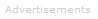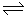# Definition of Buffers (acid-base)

What are Acid-Base Buffers?

Buffer solutions resist pH changes.A buffer is a solution that can maintain a nearly constant pH if it is diluted, or if relatively small amounts of strong acids or bases are added.

A buffer solution can be made by mixing a weak acid with one of its salts OR mixing a weak base with one of its salts.

A more technical way of saying this is that a buffer solution consists of a mixture of a weak acid and its conjugate base OR a weak base and its conjugate acid.

Example of How a Buffer Works

Consider a solution containing both acetic acid, CH3COOH, and acetate ions, CH3COO-. (These could alternatively be named ethanoic acid and ethanoate ions.) This solution can be made by dissolving sodium acetate (sodium ethanoate) in acetic acid (ethanoic acid).

Any strong base that is added to the solution is neutralized by the acetic acid, which acts to remove OH-:

CH3COOH (aq) + OH-(aq)CH3COO-(aq) + H2O (aq)

Any strong acid that is added to the solution is neutralized by the acetate, which acts to remove H+:

CH3COO-(aq) + H+(aq)CH3COOH (aq)

The amount of strong acid or base that a buffer can neutralize is called the buffer capacity.

Calculating the pH of Buffer Solution

Since the buffer concentration is usually high, the hydrogen ion concentration [H+] can be calculated from the equilibrium expression assuming that the concentration of the conjugate acid-base pair does not change appreciably.

Example
What is the pH of a buffer solution containing 0.100 moles of both CH3COOH and CH3COO- in 0.100 liters of water?

The equilibrium is:

CH3COOH (aq)CH3COO-(aq) + H+(aq)        Ka = 1.8 x 10-5 (at 25°C)

We use the following equation to find pH:

pH = pKa - p([conjugate base]/[acid])

Remembering that p is -log10, we can write:

pH = -log10Ka + log10([conjugate base]/[acid])

Therefore:

pH = -log101.8 x 10-5 + log10(1.00 M/1.00 M)

Therefore:

pH = 4.74

Notice that when the concentrations of the weak acid and conjugate bases are equal, the pH of the buffer solution equals pKa.

Let's say we add 10.0 ml of 1.00 M HCl to the buffer solution.

The CH3COO- ions in the buffer solution react with all of the H+ from the HCl to form CH3COOH.

The effect is that the concentration of CH3COOH increases and the concentration of CH3COO- decreases.

The equilibrium is:

CH3COOH (aq)CH3COO-(aq) + H+(aq)        Ka = 1.8 x 10-5 (at 25°C)

So:

```
[H+][CH3COO-]
Ka  =  ------------------------
[CH3COOH]

```

To calculate pH we need to find [H+], so we'll rearrange the above equation to get:

```
Ka [CH3COOH]
[H+]  =  ------------------------
[CH3COO-]

```

Since the volume of the weak acid and its conjugate base are always the same, we can modify the equation to read:

```
Ka (moles of CH3COOH)
[H+]  =  ------------------------
(moles of CH3COO-)

```

The changes in moles that take place after the addition of the HCl are summarized as follows:

 CH3COOH(aq) H+(aq) CH3COO-(aq) Initial Amount 0.1 moles (0.010 litres)(1.00 M) = 0.010 moles 0.1 moles Change in Amount + 0.01 moles -0.01 moles - 0.01 moles Equilibrium Amount 0.101 moles ? 0.09 moles

Therefore, we can now put the following values in our equation:

```
1.8 x 10-5 x (0.101 moles)
[H+]  =  ------------------------
(0.09 moles)

[H+] = 2.02 x 10-5

We know that pH = -log10[H+]

Therefore, pH = -log10(2.02 x 10-5) = 4.69```
Conclusion

Adding a small amount of strong acid to the buffer solution has barely changed the solution's pH from its original value of 4.74.Search the Dictionary for More Terms